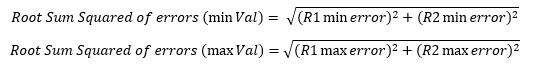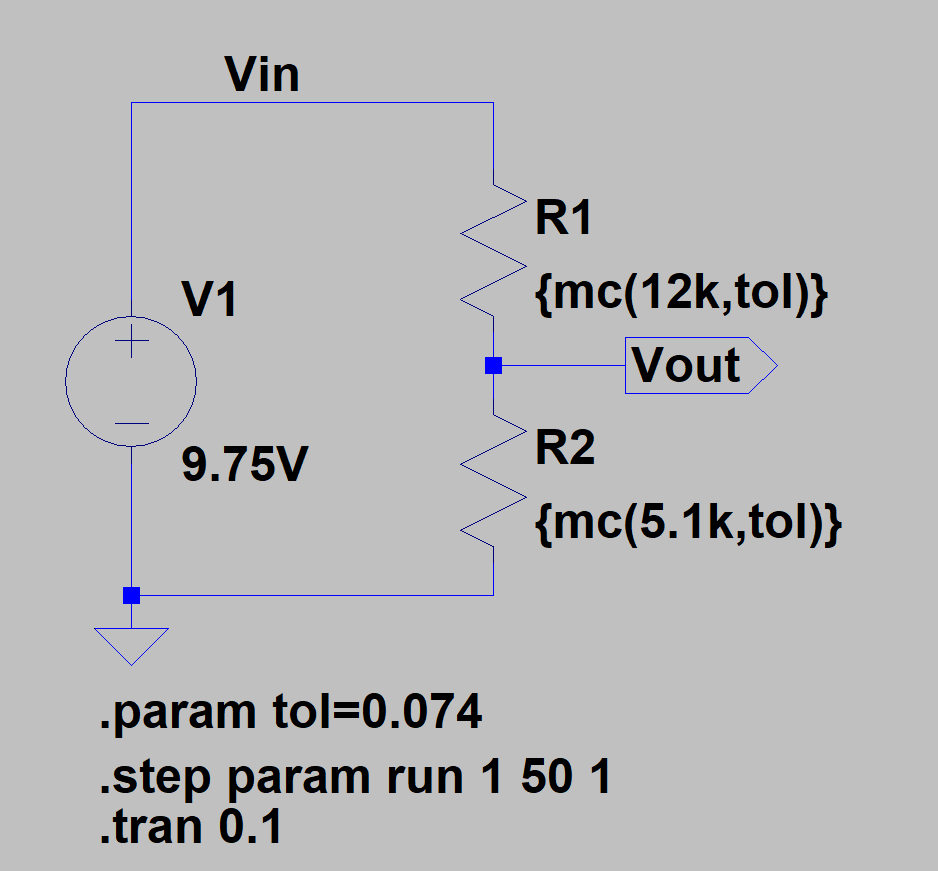# Why you really need a WCA (Worst Case Analysis)What is the worst it can happen? It’s a good question to ask when you are taking a decision. You imagine yourself going through the worst situation caused by the worst decision you can make and decide if you can go through it or not. Or maybe you need more factual data than just a thought experiment? Then you do it and find out that everything is fine and you were over exaggerating the consequences. But, were you?

It happens that when you think of the absolute worst there is only less than 5% of chances for that situation to happen (Gaussian distribution), so in your real life experiments you either have to iterate thousands of times or been really unlucky for this situation to actually happen.

This is a sort of fallacy that we can accept in day to day situations but not when designing a circuit that could potentially injure or kill someone else if it doesn’t perform to specification. As you can imagine, WCA’s are essential in the automotive, aerospace and defence industry for obvious reasons. However, they should be used whenever you are designing a circuit that has an expected shift in tolerance of components values (because you chose to use cheap components with broad tolerances), temperature or it is used for a long period of time.

It is normal than in early stages of the design, you add and take components from your analogue circuits based on “nominal” calculations as these are quicker and when tested at 25 °C in a controlled environment they give generally a good result.

For example, you have to measure the voltage of a battery present in your circuit. You are using a 9V battery but your MCU runs at 3.3V, therefore you know that a voltage divider is required to scale down the voltage level so the ADC is not saturated and the MCU does not damage.

You quickly use the voltage divider formula 3.3V*0.9 > Vout = Vin*R2/(R1+R2) and find a value for the resistors. So far so good. However, you miss the application context and didn’t notice that because you are measuring the battery you need quite an accurate reading so the MCU knows exactly what is the voltage of the battery.

Because you wanted to save costs you chose cheap 5% tolerance resistors. Apart from this, your circuit needs to work from -20 °C to 85 °C and you ignored that the resistors have a change of value depending on the temperature of the component.

When you put together the tolerance+the temperature drift error you notice that you have a range of results going from max to min that it is over the required measurement precision of the battery. At this point, you realized that you have chosen the wrong components and have to go back to your initial calculations.

As you can see from this example, it is better to design taking into account the worst case analysis situations to avoid repeating yourself (or at least reduce the number of repetitions).

## So then, what is a Worst Case Analysis?

In its simplest concept, a Worst Case Analysis is the quantification of a signal given the absolute maximum and minimum that the signal can change in the context of that circuit and its given environment in order to compare it with the circuit specifications and requirements. Wait, huh? let’s dissect that phrase for a moment:

• Quantification of a signal: these are practical analysis (although they don’t sound like it), so what we want to eventually get is a numerical value in volts, amperes, watts, Celsius and so on.
• Absolute maximum and minimum that the signal can change: we want to know how below and how above can the signal goes in respect to its nominal value.
• In the context of that circuit and its given environment: the numerical result of the WCA is a function of all the parameters that can vary in the analyzed circuit and external factors that the circuit is subjected to, such as temperature, vibration, radiation and humidity.
• In order to compare it with the circuit specifications and requirements: for a WCA to be most useful you need to compare it with a reference point. In another article (work in progress) I talk about the importance of requirements. These requirements will give the designer a target to aim and design for. With the WCA you will change components and iterate until you get into the range specified by the initial requirements.

A “nicer” definition of a Worst Case Analysis is: how does your circuit perform under extreme environmental and operating conditions when circuit components tolerances parameters are at their max and min value.

## Essential elements of a Worst Case Analysis

To perform a WCA, you need some basic information about your circuit:

1. Data about the components used and the environment: this includes components datasheets and special information required from the components supplier, device operating temperature range, device operating lifetime.
2. Circuit function and data: you need to know exactly what your circuit block does in terms of Input/Output. Think that we are treating with an analogue signal that is “processed” and changed of magnitude. With this knowledge, you need to derive the circuit transfer function. In our previous example of the voltage divider, this transfer function is the simple equation Vout = Vin*R2/(R1+R2). You also need to look for special characteristics of the circuit such as timings if its a function dealing with a signal that changes in time.
3. Circuit requirements and specifications: as said before, you need to have a reference point where you can aim your design to and compare it with.Do you want to see an example of a WCA? Would you like to have a WCA template for you to use in your projects? Download the FREE WCA Template and Example.

Your details will not be shared and you will only receive relevant content. Zero Spam

## Other types of Analysis

As we said at the beginning, the worst case analysis is the single worst situation that can happen, therefore, if you pass the conditions for this case, you can happily go to bed and sleep because you know your circuit will always perform as intended no matter how bad the situation can be (between the constraints you considered in your analysis of course).
However, sometimes this is a very pessimistic and unrealistic view, so there are other types of analysis that statistically, approach more to reality.

### Root Sum Squared Method

The RSS method takes into consideration that most of the deviations will happen at the center or nominal tolerance range (green part of the figure), this is because in the real world you can have errors that counteract each other, for example, your resistor can have a -1.2% tolerance shift from the nominal value but because the component is at 56 °C then its value shifts up.To perform a Root Sum Squared analysis you have to find out the individual contribution of each variable to the total error. So for our previous example, there are only two error contributions: R1 and R2.

First, you take your transfer function and input all the nominal values except one, for example, you use R1_max.

Then, the result from this is compared with the nominal result (all values in nominal) which gives you the proportion of error that R1_max causes to the final result.

Later you do the same with all remaining variables (R1_min, R2_max and R2_min) and apply the root sum squared formula:I understand this is a bit hard to visualize without an example, so, worry not there is a Resource available where I show you how to calculate the error with the RSS method.

### Monte Carlo Analysis

The Monte Carlo Analysis uses computer power for a repeated random sampling mathematical model (the transfer function) to generate simulation data. From many iterations, the simulation can then find out where most of the values will be, given a range of tolerances. This is best explained with an example:

From our previous voltage divider example, we look into a 9V battery datasheet (Duracell 6LR61) and we can see that the max voltage when the battery is new and it is only discharging 2mA is around 9.75V:We select this as our input voltage because we want to make sure with our simulation that we are below 0.9*3.3V = 2.97V. Then, because we want to save cost we select 5% tolerance resistors with a 300ppm/ °C temperature drift.

We select our resistors using the previous transfer function so when the battery is at 9.75V we have an output of around 2.97V.

The selected resistors are 5.1K and 12K. We can now calculate the tolerance error from the temperature drift: (5100/1M)*300*(85-25+20) = (122.4ohms*100)/5100ohms = 2.4%.

So our total tolerance will be 5+2.4 = 7.4% (here we haven’t taken into account factors such as ageing and post solder drift).

With the following values R1=12Kohms, R2=5.1Kohms, Vbat_max=9.75V and Rtol=7.4% we open LTSpice to perform our simulation:From the graph above you can see the WC values near the top and bottom and the two groups were most of the values will realistically be located.

Analyzing the graph we can see that there is a fair amount of values that will be above 2.97V, meaning that if we want to achieve this voltage level, we will have to lower the resistor tolerance.

## Conclusion

In this article, we have analyzed the importance of making a WCA of your circuit at the early design stages so you don’t have to come back later to the drawing board and change your components because the design doesn’t meet the requirements criteria. We have also looked into different tolerance analysis methods that can help you to see if it is really required to change that component based on the fact that it might be almost statistically impossible for that WC condition to happen. In general, I follow this method with my designs:

### Perform a WCA

1. If it passes easily, look to optimize the circuit by choosing less accurate components. This way you might save a bit of money
2. If it just passes, then it is OK
3. If it doesn’t pass by around 30%, look into doing an RSS or Monte Carlo Analysis and determine if it is really needed to change the components
4. If its above 30% of its required parameter, change the components

Do you know any other method to analyse circuit tolerances? Have you done any WCA before? Share your thoughts below 🙂

## References:

Posted in: Product Development